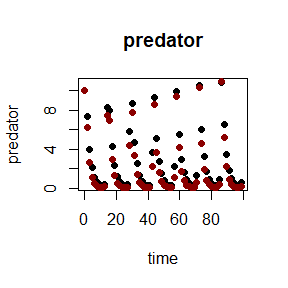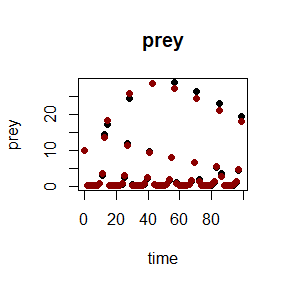# How to use paropt

The package paropt is build in order to optimize parameters of ode-systems. Thus, the aim is that the output of the integration matches previously measured states. The user has to supply an ode-system in the form of a Rcpp-function. The information about states and parameters are passed via text-files. Additional information such as e.g. the relative tolerance are passed in R. This vignette uses a predator-prey system as example to show how the package can be used.

## The ode-system

The ode system should be a Rcpp-function with a specific signature. The function is called very often, thus using a R function is not advisable. The name of the function is free to choose. The following parameters have to be passed: a double t, a std::vector params, and a Rcpp::NumericVector y. The first entry defines the time point when the function is called. The second argument defines the parameter which should be optimized. Notably, the parameters are in the same order as in the text-files containing the start-values and the information about the boundaries. The last argument is a vector containing the states in the same order as defined in the text-file containing the state-information. Thus, it is obligatory that the state-derivates in the ode-system are in the same order defined as in the text-file. Furthermore, it is mandatory that the function return a Rcpp::NumericVector with the same dimension as the input vector containing the states. Naturally, the vector should contain the right hand side of the ode-system.

#include <Rcpp.h>

// [[Rcpp::export]]
Rcpp::NumericVector ode_system(double t, std::vector<double> params,
Rcpp::NumericVector states) {

// set dimension of vector which should be returned
Rcpp::NumericVector states_deriv(2); //two states in the example above (prey and predator)

// store parameters in variables
double a = params;
double b = params;
double c = params;
double d = params;

// store states in variables
double predator = states;
double prey = states;

// the actual ode-system
double ddtpredator = states_deriv = predator*c*prey - predator*d;
double ddtprey = states_deriv = prey*a - prey*b*predator;

return states_deriv;
}

## State-input

The information of the states, have to be supplied as textfiles tab seperated. The delimiter has to be a '.'. It is possible to use scientific notations ('.', '+', '-', 'e', 'E' and naturally all digits are allowed). It is compulsory that the first column has the name time. This column contains the independent variable across the solver integrate (it has not to be the time. In this document it is always called time). It is followed by the information of the states at the specific time points. It is pivotal that the order of the states is the same as in the ode-system in order to correctly calculate the error. If a state is not availabe at all timepoints use NA in order to ignore this state at the specified timepoint for calculating the error (see Table1). However, the first line below the header cannot contain NA due to the fact that in this line the start values for the solver are defined.

Table1: Possible input for states.
time predator prey
0 10.00 10.000
2 2.13 0.022
4 0.23 3.023
6 4.30 0.028
8 0.37 5.000
10 8.60 0.330

## Types of parameters

There exists two different types of parameters: constant and variable parameters. For instance (see Table2) parameters p1, p2 and p3 are variable whereas p4 is constant during the entire time from t = 0 to t = 10.

Table2: Possible input for parameters: start values, lower- and upper-bounds
time p1 p2 p3 p4
0 0.8699751 2.8340258 0.7183206 0.3800352
2 1.1791593 1.9823934 0.4456933 NA
4 1.7612748 1.8873421 0.7928573 NA
6 2.7338026 0.1853588 0.5479293 NA
8 0.6848776 0.6179237 0.7458567 NA
10 2.7053301 0.5296703 0.9927155 NA
time p1 p2 p3 p4
0 0.1 0 0.1 0
2 0.1 0 0.1 NA
4 0.1 0 0.1 NA
6 0.1 0 0.1 NA
8 0.1 0 0.1 NA
10 0.1 0 0.1 NA
time p1 p2 p3 p4
0 3 3 1 1
2 3 3 1 NA
4 3 3 1 NA
6 3 3 1 NA
8 3 3 1 NA
10 3 3 1 NA

In the predator-prey example followed here all parameters which should be optimized are constant (see tables below).

Table3: parameter-input for predator-prey-model: start values, lower- and upper-bounds
time a b c d
0 1 0.5 0.2 0.25
time a b c d
0 0.8 0.3 0.09 0.09
time a b c d
0 1.3 0.7 0.4 0.7

The start-values, lower-bounds and upper-bounds for the parameters have to be supplied as textfiles which is tab seperated. The delimiter has to be a '.'. It is possible to use scientific notations ('.', '+', '-', 'e', 'E' and naturally all digits are allowed). As for the states it is mandatory that the first column has the name time. This column contains the independent variable across the solver integrate (it has not to be the time) The start-values have to be between the lower and upper boundary. Naturally, the lower-bounds have to be smaller than the upper-bounds.

## What happens during one evaluation of a parameterset

During the optimization the optimizer creates a bunch of possible solutions which consists of the four different values for a, b, c and d. Each solution is passed to the ode-solver which integrate along the time and return the states at the time-points specified in the text-file containing the state-information. The in silico solution is compared to the measured states in order to evaluate the parameterset. The error used is the sum of the absolute differences, between in silico and measured states, divided by the number of timepoints.

During the integration the ode-solver can reach a timepoint t where no information for the parameter is measured. For instance in table 2 the timepoint 1.5 is not defined for the parameters p1, p2 and p3. In this case the parameters have to be interpolated. This is conducted using a Catmull-Rom-Spline. The parameter-vector passed to the ode-system contains already the splined parameters at timepoint t. Thus, using the table above (see Table2) the parametervector could contain for instance at timepoint t = 1.5 (p1 = 1.11, p2 = 1.99, p3 = 0.66, p4 = 0.38) (for the start-values). Due to that, the parameters passed to the ode-system are in the same order as defined in the text-files but already interpolated.

## finally optimize the parameters

When you have the four textfiles defining the states, parameter start-values, lower- and upper-bounds and the ode-system you can start running the optimization.

path <- system.file("examples", package = "paropt")
library(paropt)
#Rcpp::sourceCpp(paste(path,"/ode.cpp", sep = ""))
#if you want compile ode-system on your system (already precompiled in package)
time <- df\$time
param_start <- paste(path, "/start.txt", sep = "")
param_lb <- paste(path, "/lb.txt", sep = "")
param_ub <- paste(path, "/ub.txt", sep = "")
states <- paste(path, "/states_LV.txt", sep = "")
state_output <- paste(tempdir(), "/final_stateoutput.txt", sep = "")
par_output <- paste(tempdir(), "/optimized_params.txt", sep = "")
set.seed(1)
optimizer(time, paropt:::ode_example, 1e-6, c(1e-8, 1e-8),
param_start, param_lb, param_ub,
states, npop = 40, ngen = 200, error = 1,
state_output, par_output, "bdf")
df_in_silico <- read.table(paste(tempdir(), "/final_stateoutput.txt", sep = ""), header = TRUE)## The arguments for function optimizer()

optimizer(time, ode, 1e-6, c(1e-8, 1e-8),
param_start, param_lb, param_ub,
states, npop = 40, ngen = 200, error = 1,
state_output, par_output, "bdf")

The first argument is the time-vector containing either the same information as in the time-column defined in the text-file containing the states (see Table1) or only a subset (it can only be shorted at the end).
It is mandatory to start with the first entry of the time-column, however it is possible to stop at a certain time-point before the last one. Thus, it is possible to optimize only a part of the problem. Next argument is the compiled ode-system (e.g. see code above which is compiled using the Rcpp::sourceCpp('odesystem.cpp') code in R). The relative tolerance and the absolute tolerances are used by the ode-solver. Next the textfiles for the start-values for the parameters (used for test-integration), the lower- and upper-bounds and the states are defined. In order to optimize the parameters a particle swarm optimizer (PSO) is used. Therefore, the number of particles (npop = 40 is a good starting point for many problems) and the number of generations (ngen) have to be passed to the function. The number of generations defines the maximum number of generations the PSO should run. However, if the PSO finds a suitable parameterset which posess an error below or equal a threshold defined by the user it stops. This threshold is defined by the error-argument. Next two names of (probably best non-exisiting) textfiles are passed. Using this names the function creates two textfiles. The first one containing the state-output of the integration using the optimized parameters and the second one contains the optimized parameters itself. The last argument defines the typ of solver to be used. There exists four different solver: 'bdf', 'ADAMS', 'ERK' and 'ARK. All solvers are part of the SUNDIALS projexct. For details see 'https://computing.llnl.gov/projects/sundials' and Hindmarsh et al. (2005). The bdf solver is the backward differential formular solver of CVODE and is best used for stiff problems. It uses a dense matrix module (SUNDense_Matrix) and the corresponding nonlinear solver (SUNLinsol_Dense). The ADAMS solver is the ADAMS-MOULTON solver of CVODE and is most suitable for non-stiff problems. The 'ERK' solver is an explicite Runge-Kutta solver which is also as the ADAMS-MOULTON solver used for non-stiff-problems. The 'ARK' solver is a fully implicte Runge-Kutta-solver which uses the same matrix and nonlinear solver module as 'bdf'. The integration itself occures for all four solvers in a for-loop using the 'CV_NORMAL' step-function. If integration for a specific parameterset is not possible the error is set to 1.79769e+308 (which is the maximum of a double).

If you want to test a specific parameterset just call the function ode_solving. The function requires the same parameter as optimizer. Naturally, the arguments for the PSO, the error-threshold as well as the parameter lower- and upper-bounds are not needed.

## Particle swarm optimizer (PSO)

This PSO-implementation is a modified version of 'https://github.com/kthohr/optim' (for a general overview see Sengupta, Basak, and Peters (2018)). The PSO has several key features. First of all a bunch (number of particles defined by user) of possible parametersets is created within the boundaries defined by the user. Each parameterset is called a particle. All particles together are called the swarm. Each possible parameterset is passed to the solver which integrates the system. The result is used to calculate the error. Thus, each particle has a current solution and a current personal best value. Furthermore, each particle possess a neighberhood which consists of 0-3 other particles (for details see D. Akman, Akman, and Schaefer (2018)). The PSO uses a combination of its history (personal best value) and the information of the best particle of the neighberhood to change its current value. Notably, in this package a randomly adaptive topology is used. This means, that the neighberhood is recalculated each time when the global best solution (best solution of the entire swarm) has not improved within one generation.